# Design a Crystal Oscillator to Match Your Application

### Abstract

Quartz crystals are mechanical resonators with piezoelectric properties. The piezoelectric properties (electric potential across the crystal is proportional to mechanical deformation) allow their use as electrical circuit elements. Crystals are widely used as resonant elements in oscillators due to their high quality factor (QF), excellent frequency stability, tight tolerance, and relatively low cost. This tutorial explains the primary design considerations to be addressed in a design of a simple crystal oscillator using AT-cut crystals. The basic qualities of a crystal oscillator and factors that can affect their performance in a variety of applications are described. The topics discussed here are the compilation of issues encountered over a decade of design and applications for ISM-band radios. These topics include load capacitance, negative resistance, startup time, frequency stability versus temperature, drive-level dependency, crystal aging, frequency error, and spurious modes.

A similar version of this article appears on Electronic Design, September 07, 2012.

### Basics of a Crystal Model

Quartz crystals are modeled electrically as a series RLC branch in parallel with a shunt capacitance (Figure 1). The series RLC branch, often called the motional arm, models the piezoelectric coupling to the mechanical quartz resonator. The shunt capacitance represents the physical capacitance formed by both the parallel plate capacitance of the electrode metallization and the stray package capacitance.The model shown in Figure 1 applies to fundamental-mode operation. Similar models also apply for overtone operation of crystal resonators. The overtone models include additional series RLC branches in parallel with the elements shown in Figure 1. The additional overtone RLC series branches have resonance frequencies near odd multiples of the fundamental series resonance frequency.

For crystals operating in the fundamental mode with a frequency range of 5MHz to 30MHz, typical values of the circuit elements are:

C1 2fF to 20fF (motional capacitance)
R1 10Ω to 150Ω (equivalent series resistance, ESR)
L1 determined by C1 and the operating frequency (motional inductance)
C0 0.5pF to 5pF (shunt capacitance)

Where the motional elements are the electrical analog of the mechanical resonance and the piezoelectric properties of the crystal. The ESR models the losses of the mechanical resonance.

For a series RLC circuit, one with no external driving voltage, the sum of all voltages results in the following differential equation:

L × dI/dt + I × R + (1/C) × ∫I × dt = 0

By definition, I can be replaced with dQ/dt, yielding:

L × d²Q/dt² + R × dQ/dt + Q/C = 0

Or

d2Q/dt² + (R/L) × dQ/dt + Q/(L × C) = 0

Which is of the form d2Q/dt² + (ω0/QF) × dQ/dt + Q × ω0² = 0.

This yields the well-known result for RLC circuits: the natural frequency, ω0, is the square root of the inverse of the product of inductance and capacitance.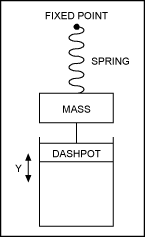The mechanical model of the crystal consists of a mass; a spring with associated spring modulus or stiffness; and a dashpot to model losses (Figure 2). The forces applied to the crystal, ignoring the fixed force and spatial offset due to gravity, result in an acceleration of the mass (Newton's Second Law of Motion). Two forces are assumed in the simple linear model, spring force and frictional force. The spring force is given by Hooke's Law, F = K × Y, where K is the spring modulus and Y is the displacement from equilibrium. The frictional loss is assumed to be proportional to the velocity of the plunger in the dashpot and the friction constant, D, of the dashpot. Equating these forces (with no external driving forces) gives:

M × d²Y/dt² + D × dY/dt + K × Y = 0

Or

d²Y/dt² + (D/M) × dY/dt + Y × (K/M) = 0

Which is of the form d²Y/dt² + (ω0/QF) × dY/dt + Y × ω0² = 0.

The resulting natural frequency of the mechanical system must equal the natural frequency of the electrical system. This yields:

ω0 = √(1/(L × C) = √(K/M)The mass of a cubic- or cylindrical-shaped quartz resonator with electrode metallization on opposing faces of the narrowest dimension is proportional to the product of the electrode area and the spacing between the electrodes (i.e., the narrowest dimension or thickness), as shown in Figure 3.

M ~ A × T

Where A is the electrode area and T is the thickness.

The spring modulus of the same cubic-shaped quartz resonator is proportional to the product of the electrode area and the inverse of the thickness.

K ~ A/T

From this, the natural frequency of the mechanical system is independent of electrode area and proportional to the inverse of the thickness as:

ω0 = √(K/M) ~ √(A/(T × A × T) = √(1/T²) = 1/T

Of the many possible crystal-resonator options, AT-cut crystals are popular for their temperature-coefficient characteristics and manufacturing repeatability. For AT-cut crystals the mechanical resonance is a shear mode, as shown in Figure 4. In this mode of operation the center of gravity moves both vertically and horizontally. Thus, the preceding analysis is a one-dimensional approximation, useful for qualitative understanding of the mechanical resonance of an AT-cut crystal.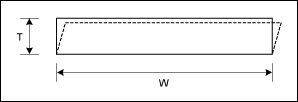From a parallel circuit perspective, the overall electrical impedance of the crystal will be inversely proportional to the electrode area, as a larger electrode area is equivalent to multiple smaller electrode area crystals in parallel. Thus, the series resistance and motional inductance will be inversely proportional to the electrode area; the motional capacitance and the parallel plate portion of the shunt capacitance will be proportional to the electrode area. The shunt capacitance and the motional capacitance have a linear relationship, as they are both proportional to the electrode area for the unpackaged crystal, usually known as a crystal blank. The relationship would be proportional if the parasitic shunt capacitance of the package was negligible and if the shunt capacitance parallel plate fringing fields were negligible.

The following is a list of design trade-offs based on the preceding analysis:

1. Smaller crystal electrode areas are attractive for lower cost and perhaps smaller package size. However, this smaller area increases series resistance, which slows startup time (see following Startup Time section) and can prevent oscillation.
2. Larger crystal electrode areas lower series resistance. However, this larger area increases the shunt capacitance which then lowers the active circuit negative resistance (see Negative Resistance section below), which, in turn, also slows startup time and can prevent oscillation. The larger crystal electrode area increases the motional capacitance. With a larger motional capacitance comes greater sensitivity to frequency shift due to external capacitive loads, or frequency "pulling" (see Load Capacitance section below).

Many crystal oscillators operate at the parallel resonance point of the crystal and the applied load capacitance. The load capacitance is defined to be the effective capacitance, external to the crystal package, applied between the terminals of the crystal as seen in Figure 5. Crystal manufacturers specify a given load capacitance along with a frequency of operation. Operation with a load capacitance that differs from the manufacturer's specified load capacitance results in an oscillation frequency error with respect to the manufacturer's specified frequency. The frequency error is due to capacitive "pulling" of the crystal. This can be demonstrated by combining the shunt and load capacitances in parallel, and then combining this summed shunt plus load capacitance in series with the motional capacitance to form the overall effective capacitance.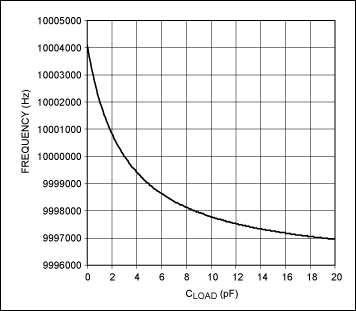### Negative Resistance

Pierce or Colpitts topology oscillators are generally used in conjunction with a crystal to generate time or frequency references. Both topologies are referred to as a "three-point oscillator". The general forms are shown in Figures 7 and 8. Note that the three points A, B, and C are identical for both topologies, except for the AC-ground point.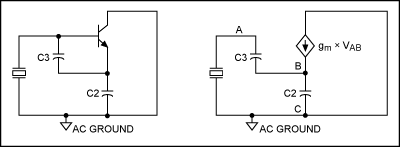To determine the impedance presented to the crystal by the transconductor (usually MOSFET or a bipolar junction transistor, but in some cases a JFET or even a vacuum tube) and capacitors C3 and C2, we can replace the crystal with a current source that drives current from point A to point C in the Pierce oscillator equivalent circuit (Figure 9). From this:

VA = -Z3 × I

Where Z3 = 1/(j × ω × C3).

VC = Z2 × I - Z2 × gM × VA = Z2 × I + Z2 × gM × Z3 × I = I × (Z2 + gM × Z3 × Z2)

and gM is the small signal change in collector current per change in base to emitter voltage for a bipolar junction transistor (gM = ΔIC/ΔVBE), or the small signal change in drain current per change in gate to source voltage for a MOSFET (gM = ΔID/ΔVGS).

Where Z2 = 1/(j × ω × C2).

VCA = VC - VA = I × (Z3 + Z2 + gM × Z3 × Z2)

ZIN = VCA/I = Z3 + Z2 + gM/(C3 × C2 × (j × ω)²) = Z3 + Z2 - gM/(C3 × C2 × ω²)Since ZIN is the impedance presented to the crystal by two capacitors and the transconductor, then the impedance presented to the crystal is effectively the series combination of C3 and C2 in series with a negative resistance. Note that this allows for ease of setting the load capacitance of the crystal by appropriate choice of C3 and C2, independent of transconductance.

This analysis would suggest that any arbitrary negative resistance for driving the crystal could be synthesized with appropriate transconductance and capacitor selection for a three-point oscillator. This is true in the absence of any stray capacitance between nodes A and C. In reality, however, some stray capacitance will always exist between nodes A and C. More importantly, the shunt capacitance of the crystal will always reduce the effective negative resistance presented to the RLC motional branch of the crystal.

To assess the effects of the crystal shunt capacitance on the three-point oscillator, see Figure 10.Returning to the equation for the input impedance of the three-point oscillator:

ZIN = Z3 + Z2 + gM × Z3 × Z2

And placing this impedance (ZIN) in parallel with CSHUNT:

ZAPPLIED = [1/ZSHUNT + 1/(Z3 + Z2 + gM × Z3 × Z2)]-1

ZAPPLIED = [(Z3 + Z2 +Z SHUNT + gM × Z3 × Z2)/(Z3 × ZSHUNT + Z2 × ZSHUNT + gM × Z3 × Z2 × ZSHUNT)]-1

ZAPPLIED = (Z3 × ZSHUNT + Z2 × ZSHUNT + gM × Z3 × Z2 × ZSHUNT)/(Z3 + Z2 +ZSHUNT + gM × Z3 × Z2)

Replacing the generic impedances with capacitive impedances and taking the real part of ZAPPLIED, the negative impedance presented by the three-point oscillator to the RLC motional branch of the crystal is:

Re{ZAPPLIED} = -(gM × C3 × C2)/[ω² × (C3 × C2 + C3 × CSHUNT + C2 × CSHUNT)² + (gM × CSHUNT)²]

Taking the derivative of Re{ZAPPLIED} with respect to gM and setting the derivative equal to zero yields the transconductance gM(MIN)R, for which the minimum (largest magnitude) of negative resistance occurs:

gM(MIN)R = ω × [(C3 × C2)/CSHUNT + C3 +C2]

At gM(MIN)R the maximum magnitude of negative resistance occurs, yielding:

Re{ZAPPLIED}|MIN = -1/{2 × ω × CSHUNT × [1 + CSHUNT × (C3 + C2)/(C3 × C2)]}

The negative resistance, Re{ZAPPLIED}, has the following characteristics:

1. Is always negative.
2. The absolute value of the negative resistance drops as CSHUNT increases. (See Figures 11 and 12.)
3. The maximum achievable absolute value of the negative resistance (at gM(MIN)R) drops as CSHUNT increases. (See Figures 11 and 12.)
4. The absolute value of the negative resistance must be larger than the motional resistance of the crystal for oscillation to occur. Generally, a typical or nominal absolute value of the negative resistance should be greater than four times the motional resistance.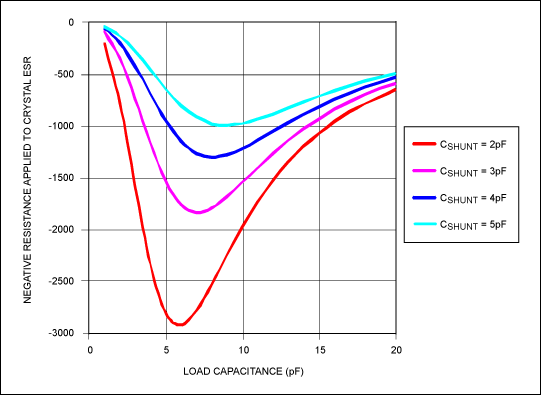Notice the strong influence of CSHUNT on both plots. Even a small increase in CSHUNT decreases the magnitude of the negative resistance in every possible configuration, especially near the peak of the negative resistance magnitude.

To apply the recommended load capacitance to the crystal and maintain higher negative resistance magnitude, it is important to keep CSHUNT small and to increase C3 and C2 to apply the necessary load capacitance. As an example, consider the following cases where the crystal load capacitance is 8pF, the operating frequency is 10MHz, the crystal CSHUNT is 2pF, the parasitic values of C3 and C2 are 8pF (due to the IC package and PCB stray capacitances), and the transconductance is fixed (due to internal IC biasing and device size) at 1mA/V.

Case 1. Use 8pF ceramic capacitors in the in the positions of C3 and C2 to load the crystal. These 8pF capacitors are in parallel with the 8pF stray capacitances for total C3 and C2 values of 16pF. This will load the crystal with 8pF, as C3 and C2 appear in series with respect to the crystal. In this case the negative resistance calculated from preceding equation for Re{ZAPPLIED} will be -627Ω.

Case 2. Use a 4pF ceramic capacitor in parallel with the crystal, as this saves the cost of one capacitor and the SMT placement of one capacitor versus Case 1. The C3 and C2 stray capacitances of 8pF each load the crystal with 4pF. The additional 4pF of shunt capacitance in parallel sum to a total of 8pF load capacitance. However, in this case the negative resistance will only be -466? due to the undesirable effects of increasing CSHUNT.

Note that Case 1 is recommended over Case 2 for crystal oscillator design because of the higher absolute value of negative resistance.

### Startup Time

The startup time of a crystal oscillator may have many different definitions depending on the type of system. The definition of startup time for a microprocessor system is often the time from initial power application to the time a stable clock signal is available. The definition of startup time for a phase locked loop (PLL) is often the time from initial power application to the time a stable reference signal is available, often settled to within an acceptable frequency offset from the final steady state oscillation frequency.

The startup time of a crystal oscillator is determined by the initial noise or transient conditions at turn-on; the small-signal envelope expansion due to negative resistance; and the large-signal final amplitude limiting due to finite power consumption.

The envelope expansion is a function only of total negative resistance and the motional inductance of the crystal. The simplified equivalent series RLC circuit will contain the motional inductance, the sum of the applied negative resistance of the three-point oscillator and the motional resistance of the crystal, and the effective series capacitance of the entire network (dominated by the motional capacitance). The following Laplace domain differential equation applies for the network (with no driving function):

s × L + R + 1/(s × C) = 0

Or

s² + s × (R/L) + 1/(L × C) = 0

The roots for this equation lie at:

(½) × {-R/L +/- √[(R/L)² - 4/(L × C)]}

Since the R/L term inside the square root is dominated completely by the 1/(L × C) term, this reduces to:

-R/(2 × L) +/- j × √[1/(L × C)]

Because the value of the net resistance R is negative, the poles of this system are in the right-half plane, and the resulting time-domain solution for this differential equation is:

V(t) = K × [e|(R/2 × L)| × t] × sin{2 × π × √[1/(L × C)] × t + Θ}

Where K is a constant related to the initial startup condition and Θ is an arbitrary phase related to the initial startup condition. (Note that the exponential expansion will be valid only for small-signal conditions, as the power available to the circuit is limited.)

The time constant for the envelope expansion is positive and proportional to the net negative resistance of the three-point oscillator and the motional resistance, and inversely proportional to the motional inductance. Due to the large motional inductance of crystals and the limited net negative resistance, crystal oscillators have very long startup times.

As an example of the envelope expansion time constant of a crystal oscillator startup, assume a crystal with 5fF motional capacitance, and an oscillator with 1500Ω negative resistance magnitude operating at 10MHz. Using the motional capacitance and the operating frequency, a motional inductance of 50.66mH can be determined by L = 1/(C × ω²). This motional inductance yields an oscillation envelope expansion time constant of τ = 2 × L/|R| = 67.55µs. Note that a trade-off exists between a smaller frequency pulling due to low motional capacitance and longer startup times due to high motional inductance, of which high motional inductance is a direct result of low motional capacitance. A mitigating factor is that smaller motional capacitances are also associated with smaller shunt capacitances, which will yield larger negative resistances and, thereby, improve startup time.

Startup time is an important design consideration in many battery-powered applications where systems are duty cycled between off and on operating states. A shorter crystal-oscillator startup time limits the wasted power in full-chip warmup times in low-power radio systems such as those employing the MAX7032 transceiver, the MAX1472 transmitter, and the MAX7058 transmitter.

### Frequency Stability Versus Temperature

The frequency shift of resonant frequency versus temperature is a function of the angle of the crystal cut versus the lattice structure of the quartz. The relative frequency shift versus temperature of AT-cut quartz crystals can be represented as a cubic polynomial:

Δf/f0 = A0 + A1(T - T0) + A2(T - T0)² + A3(T - T0

Where the coefficients A0 through A3 are functions of the angle of the quartz cut.

Frequency stability is very important in radio systems with a crystal as the system frequency reference. This is especially true for narrow-channel applications at high frequency. An example would be operation in the 25kHz channel bandwidth portion of the 863MHz to 870MHz ISM band in Europe. In these channels a frequency shift of 5kHz out of 865MHz (5.78ppm) could result in system failure or regulatory noncompliance. As can be seen in Figure 13, this is impossible to achieve even with a perfect cut-angle, zero-tolerance, zero-aging crystal over the industrial temperature range of -40°C to +85°C. For this case a radio system with an internal temperature sensor and narrow-frequency-step fractional-N synthesizer, such as the MAX7049 transmitter, can be used to compensate for known crystal frequency temperature coefficients.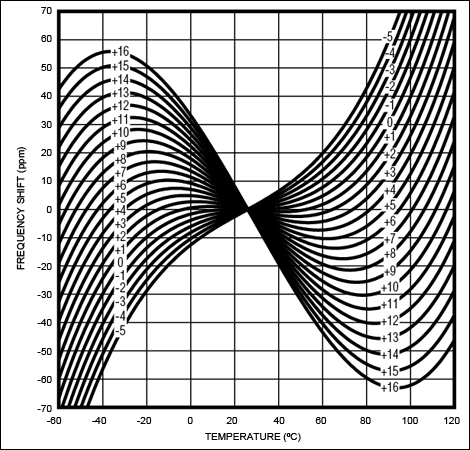### Aging

The series resonance frequency of a crystal will change slowly over time. This is known as aging. Generally a frequency change of a few parts per million occurs over a period of years. The majority of the change usually occurs over the first year or two. Aging is often attributed to a crystal mass change versus time. The aging rate accelerates at higher temperatures and at higher oscillation amplitudes.

### Compilation of Frequency Error Sources

Frequency error is due the following sources:

1. Initial tolerance, which is the manufacturer's guaranteed frequency tolerance at +25°C and with the specified load capacitance applied to the crystal
2. Frequency stability versus temperature
3. Pulling due to load capacitance variations
4. Aging

### Drive-Level Dependency

The series resistance of a crystal can rise to a level much higher than the maximum listed on the manufacturer's data sheet after some time of inactivity. The period of inactivity can range from hours to weeks. Often the condition cannot be repeated. Once the crystal has been vibrated either by an electrical or mechanical transient, the series resistance returns to the normal data-sheet limits. This is known as drive-level dependency, or DLD and sometimes referred to as "sleepy crystals," when after a period of inactivity the crystal series resistance is a function of the AC electrical drive level.

This condition is believed to be the result of additional mechanical losses due to contamination inside the crystal package. The contamination can be liquid or particulate. The liquid can be moisture which randomly condenses or freezes on the crystal below certain temperatures. The normal series resistance condition returns once the crystal vibration removes the contamination from the quartz surface. Often the contamination does not settle again (or at least not to the same degree) on the quartz surface, thus yielding unpredictable behavior after subsequent periods of inactivity.

In other cases a permanently attached particle with time and/or oscillation amplitude dependent properties, poorly adhering electrode plating, mechanical scratching, or other defects can result in DLD.

No crystal products are completely free from DLD, but higher-quality products exhibit much lower DLD, both in degree of series resistance increase and percentage of units exhibiting resistance changes.

To mitigate the problem associated with DLD the following steps can be taken:

1. Operate with large negative resistance, greater than four times the manufacturer's maximum specified series resistance. This will overcome nearly all DLD issues.
2. Purchase from higher-quality crystal vendors.
3. Pay the premium for DLD testing.

### Spurious Modes

Undesired mechanical resonances often exist near the fundamental frequency. These "spurious modes" can be modeled as additional series RLC branches in parallel with the desired fundamental frequency RLC branch in the same way that overtone operation is modeled. The spurious modes have larger losses (lesser opportunity to oscillate) than the desired mode; they usually do not cause crystal oscillator problems unless they are very low loss or the active circuitry is very weakly limited.

Generally crystal manufacturers test for spurious modes and will not ship units with low losses (i.e., greater opportunity to oscillate) at spurious resonant frequencies.

Oscillators with large negative resistances usually limit or clip during most of the oscillation cycle. During limiting the effective gain of the circuit is nearly zero. Consequently, spurious modes do not have the necessary gain to oscillate and are effectively choked by the desired large-signal oscillation. In some cases with lower negative resistance or circuits with soft limiting, enough gain exists during the entire desired oscillation cycle to support a secondary undesired oscillation. The resulting coexistence of multiple oscillations can wreak havoc with phase-frequency-detectors in PLLs and other circuits.

### Conclusion

This article has explained the primary design considerations for a simple crystal oscillator. Topics relevant in other types of radio systems, such as phase noise of crystal oscillator circuits, are not limiting factors in ISM radios and are not discussed.

The author wishes to thank Ramon Cerda at Crystek Corporation for his valuable contribution to this article.

### 参考电路

1. Vittoz, Eric A., Degrauwe, Marc G. R., and Bitz, Serge, "High-Performance Crystal Oscillator Circuits: Theory and Application," IEEE Journal of Solid-State Circuits, Vol. 23, No. 3, June 1988.
2. Kreyszig, Erwin, Advanced Engineering Mathematics, Fifth Edition, John Wiley and Sons, 1983.
3. Bechmann, Rudolf, "Frequency-Temperature-Angle Characteristics of AT-type Resonators Made of Natural and Synthetic Quartz," Proceedings of the IRE, November 1956.